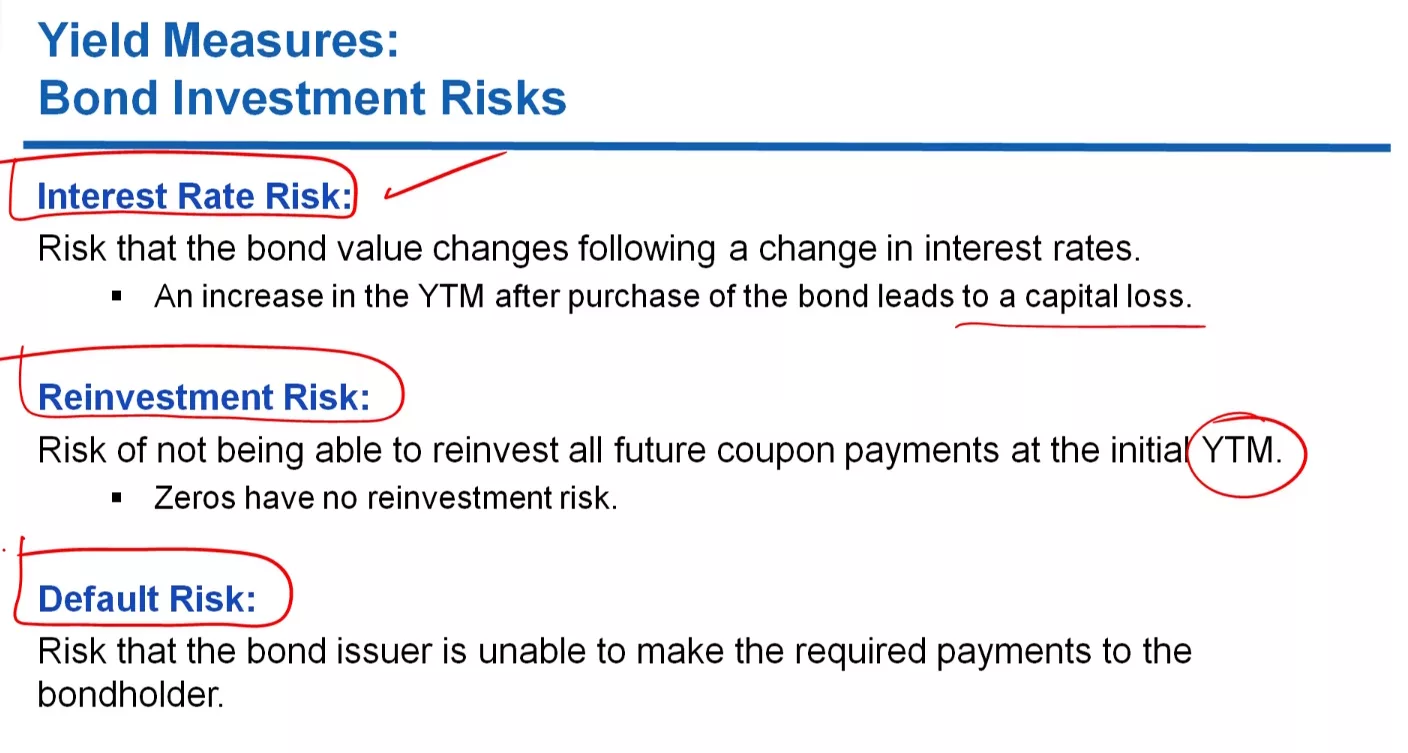# INVESTMENT RISKS IN BOND PORTFOLIOS

Jul 22, 2023Views: 250

To really understand what is the dynamic of bond pricing, we’ve been ignoring all kinds of risks associated with bond investments. But of course, in the real world, there are a number of risks that bond investors are facing on a daily basis. Let’s take a look at some of them. The first one is the interest rate risk. So the interest rate risk is the risk of having capital losses. What does that mean? The interest rate risk is the risk that the bond value changes following a change in the yields, or in interest rate. For example, suppose that you buy a bond, and then after that, the yield to maturity increases considerably.
Then, that means that your price is falling because the present value of the cash flows
is falling because the yield to maturity has increased. So if that happens, you’re going to suffer a capital loss, if the interest rate increases after the purchase of the bond price. And this kind of risk that you’re facing is called interest rate risk.
There is another kind of risk which is called the reinvestment risk. And it’s–the reinvestment risk is basically the risk that you’re facing when–it is the risk of not being able to invest all the future coupon payments at the initial yield to maturity.
So that’s called the reinvestment risk. Of course, zero-coupon bonds don’t have any reinvestment risk, right. They have–because they don’t pay any coupons, so there’s no risk of reinvesting the future coupons at a lower interest rate. And then also, importantly, but this goes well beyond the scope of this lecture, there is the default risk. What is the default risk?
It is the risk that the issuer will not make the promised payments to the bondholder.